# Subtraction

Test what do you know about the subtraction of two numbers:
a) make a difference if the minuend 4,307,288 and subtrahend is 472008
b) minuend is 4000 more than subtrahend. What's the difference?
c) the difference is 38900 less than the minuend. What is the subtrahend?

a =  3835280
b =  4000
c =  38900

### Step-by-step explanation:

$a=4307288-472008=3835280$
$b=4000=4000$
$c=38900=38900$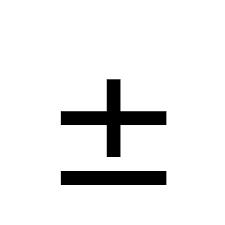Did you find an error or inaccuracy? Feel free to write us. Thank you!## Related math problems and questions:

• Pension or fraud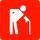Imagine that your entire working life working honestly and pay taxes and to Social Insurance (in Slovakia). You have a gross wage 900 Euros, you pay social insurance (you and your employer) monthly € 288 during 41 years and your retirement is 405 Eur
• Integers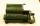May be the sum of two integers less than their difference?
• I think numberI think number.When I add 841 to it and subtract 157, I get a number that is 22 greater than 996. What number I thinking?
• RemaindersIt is given a set of numbers { 170; 244; 299; 333; 351; 391; 423; 644 }. Divide this numbers by number 66 and determine set of remainders. As result write sum of this remainders.
• Dividing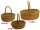When dividing the tangerines into packages of 8 or 10, one always remained. How many were there if there were more than 250 and less than 300?
• Infants water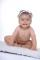The tank, which has 10 hectoliters of water for infants, went 580 liters in the morning and 340 liters in the afternoon. How many liters left in the tank?
• ConcertOn a Concert were sold 150 tickets for CZK 360, 235 tickets for 240 CZK and 412 for 180 CZK. How much was the total revenues for tickets?
• NumberDetermine unknown number if you know that difference between five times and triple of number is 42.
• Exhibition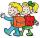The teacher paid 280 Kč for 4.A students for admission to the exhibition. How many students were at the exhibition?
• Quotient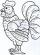Determine the quotient (q) and the remainder (r) from division numbers 100 and 8. Take the test of correctness.
• Dividing moneyVilem, Cenek, and Edita divided the money they earned by spreading the leaflet. Vilem got 240 CZK more than Cenek and twice more than Edita. Edita got 400kc less than Vilem.Why does 1 3/4 + 2 9/10 equal 4.65? How do you solve this?Today's temperature was 80 degrees, and then the temperature dropped 10 degrees. Then it dropped 15 degrees again, then the next day, the temperature went up 2 degrees. What would the temperature be?How much and how many times is 72.1 greater than 0.00721?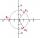Find the cube roots of 125(cos 288° + i sin 288°).How many integers are greater than 547/3 and less than 931/4?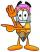By how many is the difference of numbers 8 and 34 less than its product?